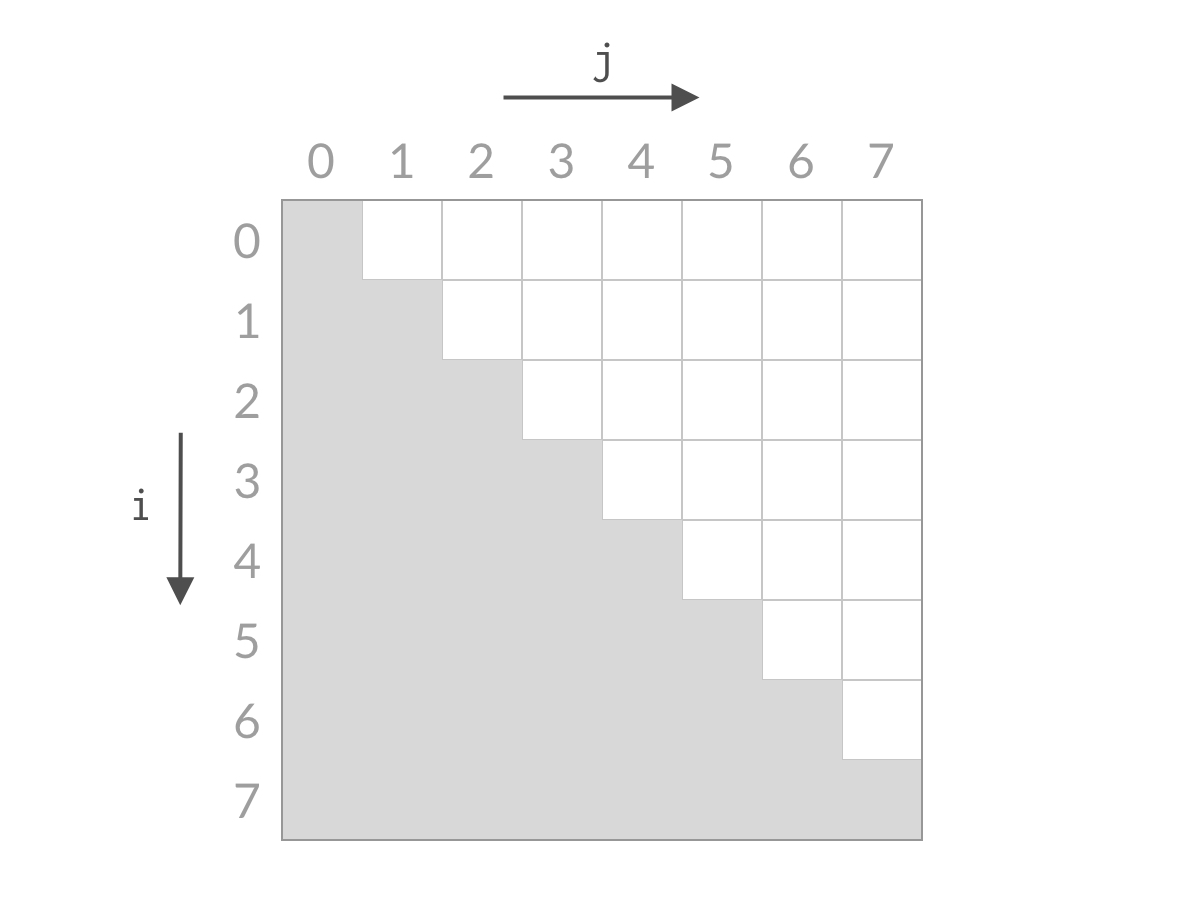# [力扣_11] 盛最多水的容器

### 题目入口：点击进入

• 暴力破解——时间复杂度O(n²)，空间复杂度O(1)
• 双指针(缩减搜索空间)——时间复杂度O(n)，空间复杂度O(1)

### 暴力破解

``````#include<stdio.h>
#include<stdlib.h>
int main()
{
int nums = { 1,8,6,2,5,4,8,3,7 };
int numsSize = 9;
int max = 0;
for (int i = 0; i < numsSize - 1; i++)
{
for (int j = i + 1, l = 1; j < numsSize; j++, l++)
{
int min = nums[i] < nums[j] ? nums[i] : nums[j];//取出最小值
int dep = min * l;
if (dep > max) max = dep;
}
}

printf("%d", max);
system("pause");
return 0;
}``````

### 双指针

``````int maxArea(int* height, int heightSize){

if(heightSize<=1)
return 0;

int max = 0;
int leng=heightSize-1;
for(int i=0,l,j=heightSize-1; leng > 0; leng=j-i)
{
int temp=height[i]<height[j]?height[i]:height[j];
if(temp==height[i])
i++;
else
j--;
temp=temp*leng;
if(temp>max)
max=temp;
}
return max;
}``````• 当前柱子是最两侧的柱子，水的宽度d为最大，其他的组合，水的宽度都比这个小
• 左边柱子较短，决定了水的高度为3，如果移动左边的柱子，新的水面高度不确定，一定不会超过右边柱子高度7
• 如果移动右边的柱子，新的水面高度一定不会超过左边柱子的高度3，也就是不会超过现在水面高度。#### 图解双指针解法原理

• i 和 j 都是合法的柱子(边界)下标，即0<=i<n，0<=j<n
• i 和 j 的左边，即 i < j## 本文链接：

https://nullcode.fun/57.html
1 + 5 =